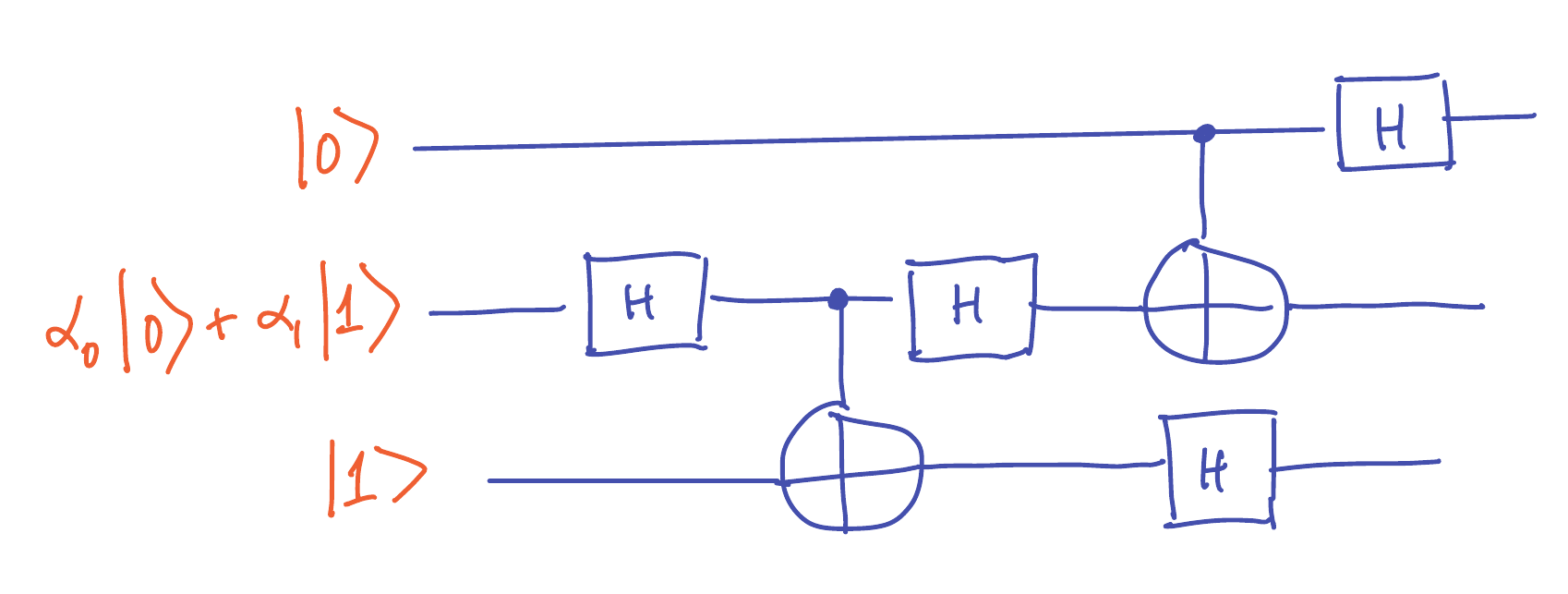# CSE 599Q: Homework #2

## Quantum circuits

Due: Sun, Oct 23 @ 11:59pm

## 1. Measuring to defeat corruption [10 pts]

Recall the 2D rotation operator

$R_{\theta} = \begin{bmatrix} \cos (\theta) & - \sin(\theta) \\ \sin(\theta) & \cos(\theta) \end{bmatrix}$

Let $\varepsilon > 0$ be some fixed constant. Consider a quantum state $\ket{\psi} \in \C^2$ and suppose that corruption from the environment ensures that over any time period $[t_1,t_2]$, the operator $R_{(t_2-t_1)\varepsilon}$ is applied to $\ket{\psi}$.

1. [2 pts] If $\ket{\psi} = \ket{0}$ initially and you don’t do any measurements, what is the state of $\ket{\psi}$ after time $T_{\mathrm{final}} \seteq \pi/(2\varepsilon)$ has passed?

2. [4 pts] Suppose you know that $\ket{\psi}$ is initially in state $\ket{0}$ or $\ket{1}$. You are allowed to measure $\ket{\psi}$ at $N$ times $t_1,t_2,\ldots,t_N \in [0,T_{\mathrm{final}}]$.

You want to maximize the probability that $\ket{\psi}$ is likely to be in its initial state at time $T_{\mathrm{final}}$. Suggest which times $t_1,t_2,\ldots,t_N$ you would choose and what measurements you would perform.

3. [4 pts] Under your scheme, analyze the probability that, despite the corruption, $\ket{\psi}$ is equal to its initial state (which was either $\ket{0}$ or $\ket{1}$) after every one of the $N$ measurements.

## 2. A quantum circuit [12 pts]

Consider the following quantum circuit that takes 3 input qubits:Note that each $H$ represents a Hadamard gate, and $\oplus$ represents a controlled not (CNOT) gate.

1. [6 pts] Determine (with proof) the state of the three qubits at the end of the circuit’s operation.

2. [6 pts] Suppose the bottom two qubits are mesured in the standard basis. Determine the probabilities of the possible outcomes, and what state the top qubit collpases to in each of the four cases.

## 3. Quantum architecture [10 pts]

SOLUTION

Suppose you have two qubits $\ket{A}$ and $\ket{B}$ and you can apply a $\mathsf{CNOT}$ gate to the pair with $\ket{A}$ as the control bit, but because of physical limitations, your system is not able to apply $\mathsf{CNOT}$ to the pair with $\ket{B}$ as the control bit. Show that if you also have the ability to apply single-qubit Hadamard gates, then you can still achieve a circuit that implements $\mathsf{CNOT}$ with $\ket{B}$ as the control bit.

## 4. Polarization detective [18 pts]

SOLUTION

The following four matrices are the single qubit Pauli operators:

$I = \begin{bmatrix} 1 & 0 \\ 0 &1 \end{bmatrix} \quad X = \begin{bmatrix} 0 & 1 \\ 1 & 0 \end{bmatrix} \quad Y = \begin{bmatrix} 0 & -i \\ i & 0 \end{bmatrix} \quad Z = \begin{bmatrix} 1 & 0 \\ 0 & -1 \end{bmatrix} \quad$

Suppose you are given a single-qubit gate $U$ and you are allowed to apply it only once. All you know is that $U \in \{I,X,Y,Z\}$.

1. [6 pts] Suppose you are allowed to evaluate $U$ on an arbitrary single qubit state $\ket{\psi} = \alpha_0 \ket{0} + \alpha_1 \ket{1}$ of your choice, and then measure in any orthonormal basis of your choice. Prove that it is impossible to choose a measurement and an input state $\ket{\psi}$ such that you can determine which Pauli operator $U$ is with certainty.

2. [4 pts] Show that the four states $(U \otimes I) \frac{1}{\sqrt{2}} \left(\ket{00} + \ket{11}\right)$ are pairwise orthogonal as $U$ ranges over $\{I,X,Y,Z\}$.

3. [8 pts] Suppose that you are allowed to evaluate $U \otimes I$ once on a 2-qubit state

$\ket{\psi} = \alpha_{00} \ket{00} + \alpha_{01} \ket{01} + \alpha_{10} \ket{10} + \alpha_{11}\ket{11},$

and then you can measure $(U \otimes I) \ket{\psi}$ in an arbitrary orthonormal basis of $\C^4$.

Show that by choosing $\ket{\psi}$ and a measurement basis appropriately, you can determine with certainty which Pauli operator is implemented by $U$.

Remark: Suppose you send a photon through two polarization filters that are each oriented either horizontally or vertically (so there are four possibilities). Then the Pauli operators represent the action of those four configurations on a photon, and the first part of the problem asks you to show that there is no measurement that can determine the configuration from passing a single photon through the filters.

The second part of the problem asks you to show that if you first entangle two photons and then send one photon through the pair of filters, then by measuring the joint pair of photons, you can actually figure out the configuration with certainty.